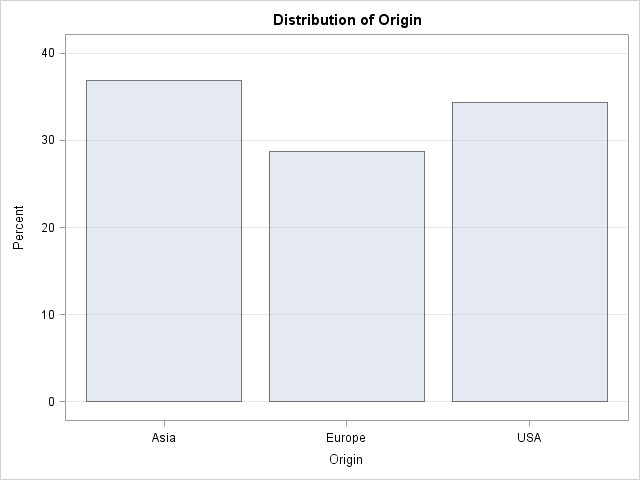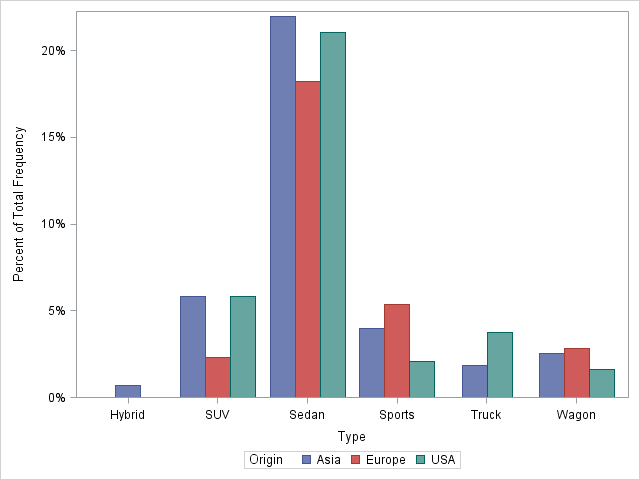# Show percentages for bar charts with PROC SGPLOT

December 3, 2012
By

 This post was kindly contributed by The DO Loop - go there to comment and to read the full post.

It seemed like an easy task. A SAS user asked me how to use the SGPLOT procedure to create a bar chart where the vertical axis shows percentages instead of counts.

I assumed that there was some simple option that would change the scale of the vertical axis from counts to percentages. After all, if you use the BARCHART statement in the GTL, you can use the STAT=PCT option to accomplish this.
Unfortunately, PROC SGPLOT in SAS 9.3 does not support the STAT=PCT option.
I checked the SAS 9.3 documentation for the VBAR statement several times, but, by golly, I didn’t see any option that sets the scale!

At last I concluded that I would need to pre-compute the percentages and use the RESPONSE= option on the VBAR statement to specify the scale of the vertical axis.

### One-way frequencies and bar charts

Assume that you want to display percentages instead of counts. If you are creating a bar chart for a one-way analysis of a categorical variable, the easiest way to visualize the categories is to use the ODS graphics in PROC FREQ. The TABLES statement supports creating a bar chart, and you can specify the scale of the vertical axis with the SCALE= option, as follows:

``` /* Frequency plot of percentages for one variable */ ods graphics on; proc freq data=sashelp.cars; tables Origin / plots=FreqPlot(scale=Percent) out=Freq1Out; /* save Percent variable */ run; ```However, sometimes you might want to use the SGPLOT procedure, especially if you want to add titles or reference lines, or otherwise change the default properties of the bar chart. In that case, you can use the information in the Freq1Out data set
that is created by the OUT= option on the TABLES statement. The Percent variable contains values in the range [0, 100].
I sometimes like to use values in the range [0,1]. The following DATA step divides by 100 and applies the PERCENTw.d format before plotting the summarized data:

``` /* use PROC SGPLOT to create a bar chart that shows percentages */ /* optional: divide by 100 and apply PERCENTw.d format */ data Freq1Out; set Freq1Out; Percent = Percent / 100; /* adjust range to [0, 1] */ format Percent PERCENT5.; run;   proc sgplot data=Freq1Out; vbar Origin / response=Percent; /* axis shows percentages instead of counts */ run; ```### Two-way frequencies and grouped bar charts

The same trick works if you want to create a grouped bar chart. As before, you can create the bar chart directly by using the ODS graphics in PROC FREQ:

``` /* Frequency plot of percentages for two variables */ proc freq data=sashelp.cars; tables Origin*Type / plots=FreqPlot(twoway=cluster scale=Percent) out=Freq2Out; run; ```The bar chart looks very similar to the bar chart that is produced by using the SGPLOT procedure and the summarized data in the Freq2Out data set:

``` /* use PROC SGPLOT to create a grouped bar chart that shows percentages */ /* optional: divide by 100 and apply PERCENTw.d format */ data Freq2Out; set Freq2Out; Percent = Percent / 100; format Percent PERCENT5.; run;   proc sgplot data=Freq2Out; vbar type /group=Origin groupdisplay=cluster response=Percent; run; ```Notice an interesting difference in the two-way (grouped) bar chart: the FREQ procedure plots empty categories, such as the category of European trucks, whereas the SGPLOT procedure does not. Of course, the biggest difference between the PROC FREQ bar charts and the PROC SGPLOT bar charts are the washed-out colors in the PROC FREQ graphs. In order to show grid lines in the background, the template for the FREQ bar chart uses semi-transparent bars, which results in the washed-out colors.

In conclusion, yes, you can use PROC SGPLOT to create a bar chart that shows percentages, but you need to pre-compute the percentages. Can you think of a different way to accomplish this task?

tags: Statistical GraphicsThis post was kindly contributed by The DO Loop - go there to comment and to read the full post.

Tags: ,

## Welcome!

SAS-X.com offers news and tutorials about the various SAS® software packages, contributed by bloggers. You are welcome to subscribe to e-mail updates, or add your SAS-blog to the site.## SponsorsDear readers, proc-x is looking for sponsors who would be willing to support the site in exchange for banner ads in the right sidebar of the site. If you are interested, please e-mail me at: tal.galili@gmail.com

## Recent Posts

SAS and all other SAS Institute Inc. product or service names are registered trademarks or trademarks of SAS Institute Inc. in the USA and other countries. ® indicates USA registration.